Question

# The method of tree ring dating gave the following years A.D. for an archaeological excavation site....

The method of tree ring dating gave the following years A.D. for an archaeological excavation site. Assume that the population of x values has an approximately normal distribution.

 1271 1229 1320 1187 1268 1316 1275 1317 1275

(a) Use a calculator with mean and standard deviation keys to find the sample mean year x and sample standard deviation s. (Round your answers to the nearest whole number.)

 x = A.D. s = yr

(b) Find a 90% confidence interval for the mean of all tree ring dates from this archaeological site. (Round your answers to the nearest whole number.)

 lower limit A.D. upper limit A.D.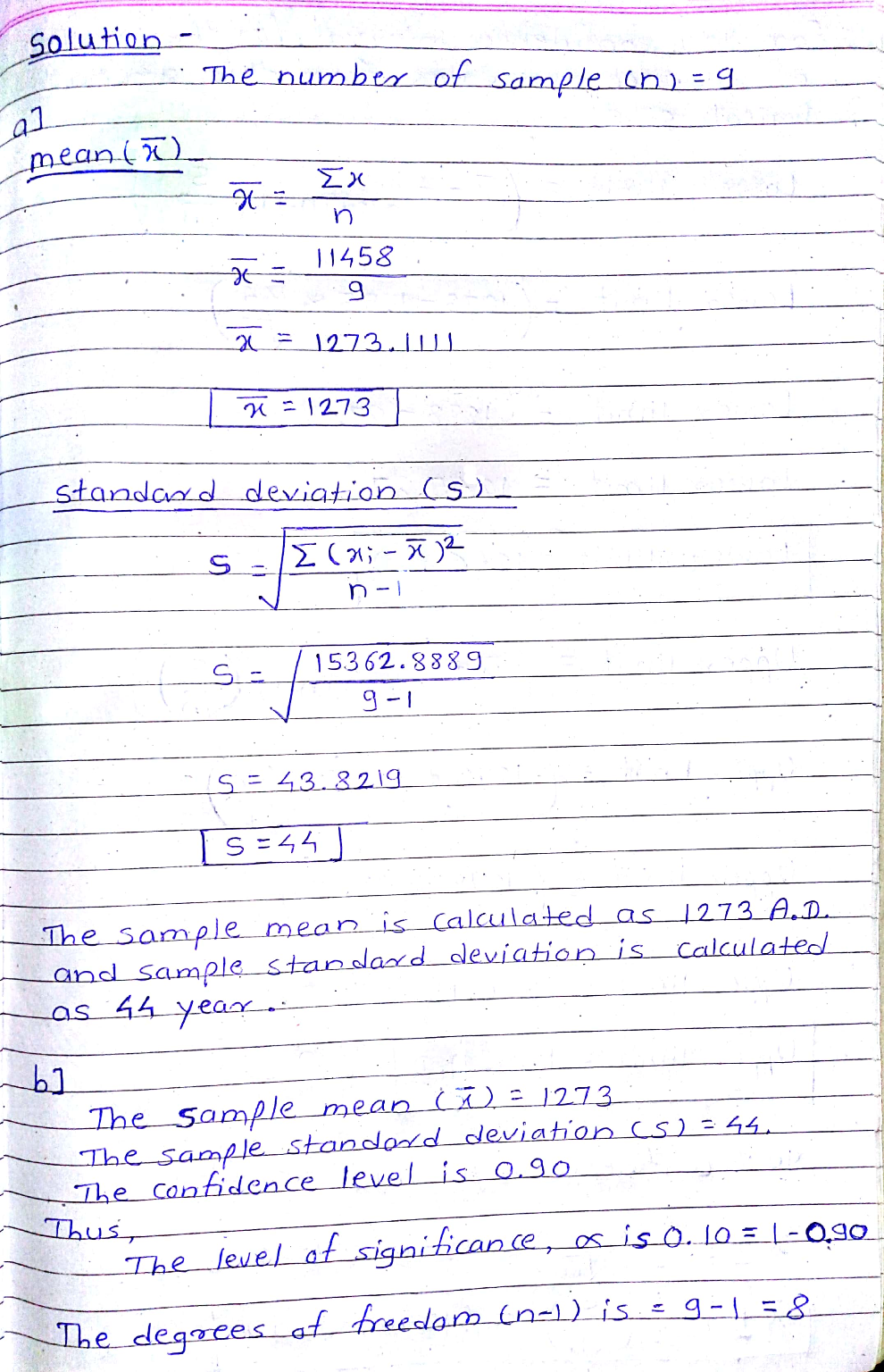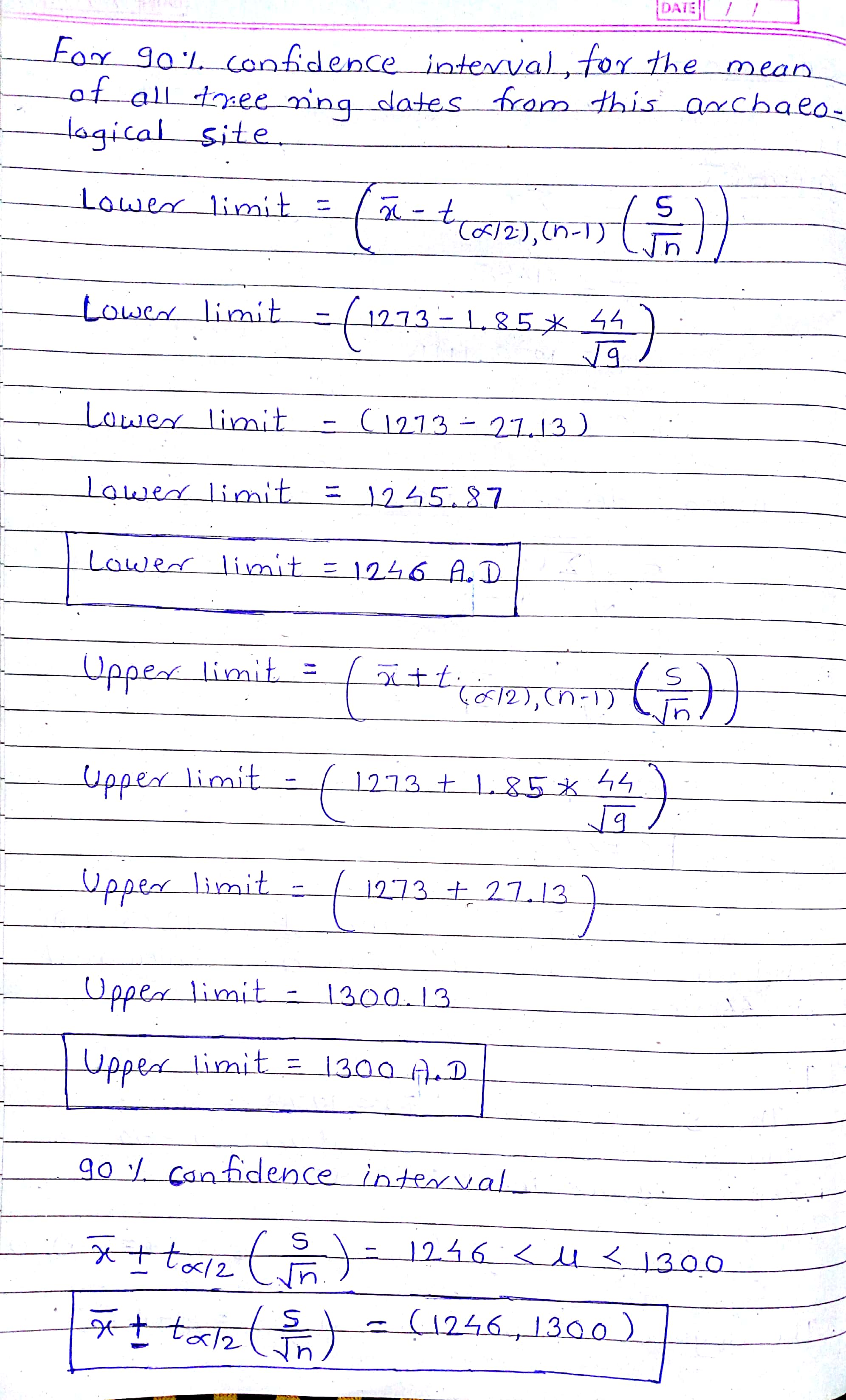#### Earn Coins

Coins can be redeemed for fabulous gifts.

Similar Homework Help Questions
• ### The method of tree ring dating gave the following years A.D. for an archaeological excavation site....

The method of tree ring dating gave the following years A.D. for an archaeological excavation site. Assume that the population of x values has an approximately normal distribution. 1250 1271 1285 1320 1268 1316 1275 1317 1275 (a) Use a calculator with mean and standard deviation keys to find the sample mean year x and sample standard deviation s. (Round your answers to the nearest whole number.) x = A.D. s = yr (b) Find a 90% confidence interval for...

• ### The method of tree ring dating gave the following years A.D. for an archaeological excavation site....

The method of tree ring dating gave the following years A.D. for an archaeological excavation site. Assume that the population of x values has an approximately normal distribution. 1201   1229   1264   1257   1268   1316   1275   1317   1275 (a) Use a calculator with mean and standard deviation keys to find the sample mean year x and sample standard deviation s. (Round your answers to the nearest whole number.) x =   A.D. s =   yr (b) Find a 90% confidence interval for...

• ### The method of tree ring dating gave the following years A.D. for an archaeological excavation site....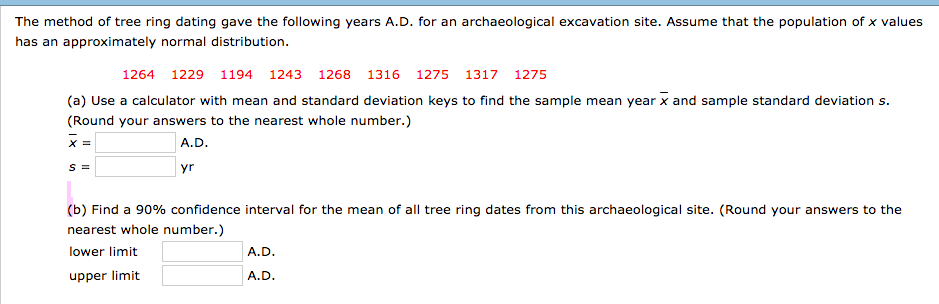The method of tree ring dating gave the following years A.D. for an archaeological excavation site. Assume that the population of x values has an approximately normal distribution. 1264 1229 1194 1243 1268 1316 1275 1317 1275 (a) Use a calculator with mean and standard deviation keys to find the sample mean year x and sample standard deviation s. (Round your answers to the nearest whole number.) A.D yr (b) Find a 90% confidence interval for the mean of all...

• ### The method of tree ring dating gave the following years A.D. for an archaeological excavation site....

The method of tree ring dating gave the following years A.D. for an archaeological excavation site. Assume that the population of x values has an approximately normal distribution. 1243 1208 1320 1201 1268 1316 1275 1317 1275 (a) Use a calculator with mean and standard deviation keys to find the sample mean year x and sample standard deviation s. (Round your answers to the nearest whole number.) x = A.D. s = yr (b) Find a 90% confidence interval for...

• ### The method of tree ring dating gave the following years A.D. for an archaeological excavation site....

The method of tree ring dating gave the following years A.D. for an archaeological excavation site. Assume that the population of x values has an approximately normal distribution. 1285 1236 1236 1271 1268 1316 1275 1317 1275 (a) Use a calculator with mean and standard deviation keys to find the sample mean year x and sample standard deviation s. (Round your answers to the nearest whole number.) x = _____A.D. s = _____yr (b) Find a 90% confidence interval for...

• ### The method of tree ring dating gave the following years A.D. for an archaeological excavation sit...

The method of tree ring dating gave the following years A.D. for an archaeological excavation site. Assume that the population of x values has an approximately normal distribution. 1229 1194 1229 1201 1268 1316 1275 1317 1275 (a) Use a calculator with mean and standard deviation keys to find the sample mean year x and sample standard deviation s. (Round your answers to the nearest whole number.) x =   A.D. s =   yr (b) Find a 90% confidence interval for...

• ### The method of tree ring dating gave the following years A.D. for an archaeological excavation site....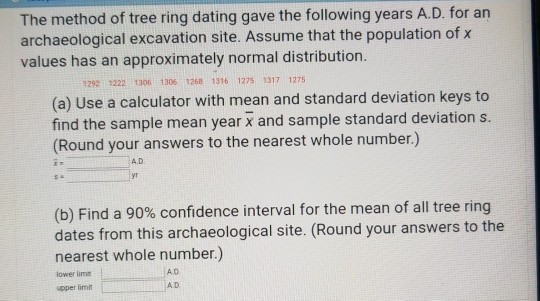The method of tree ring dating gave the following years A.D. for an archaeological excavation site. Assume that the population of x values has an approximately normal distribution. 1292 1222 1306 1306 1268 1316 1275 1317 1275 (a) Use a calculator with mean and standard deviation keys to find the sample mean year x and sample standard deviation s. (Round your answers to the nearest whole number.) A.D yt (b) Find a 90% confidence interval for the mean of all...

• ### The method of treening dating gave the following years A.D. for an archaeological excavation site. Assume...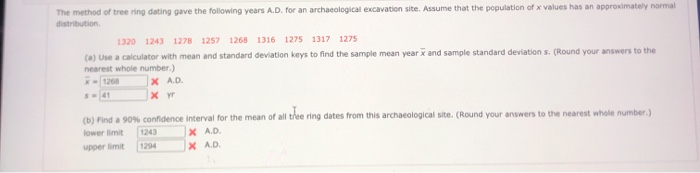The method of treening dating gave the following years A.D. for an archaeological excavation site. Assume that the population of x values has an approximately normal distribution 1320 1243 1278 125 1268 1316 1275 1317 1275 (a) Use a calculator with mean and standard deviation keys to find the sample mean year and sample standard deviation s. (Round your answers to the nearest whole number.) X AD. хуг (b) rind a 90% confidence interval for the mean of all the...

• ### QUESTION #1 The method of tree ring dating gave the following years A.D. for an archaeological...

QUESTION #1 The method of tree ring dating gave the following years A.D. for an archaeological excavation site. Assume that the population of x values has an approximately normal distribution. 1320 1243 1278 1257 1268 1316 1275 1317 1275 (a) Use a calculator with mean and standard deviation keys to find the sample mean year x and sample standard deviation s. (Round your answers to the nearest whole number.) x = ________ A.D. s = ______ yr (b) Find a...

• ### The method of tree ring dating gave the following years A.D. for an archaeological excavation site

The method of tree ring dating gave the following years A.D. for an archaeological excavation site. Assume that the population of values has an approximately normal distribution. 1264 1264 1194 1306 1268 1316 12751317 1275 (a) Use a calculator with mean and standard deviation keys to find the sample mean year and sample standard deviations. (Round your answers to the nearest whole number.) (b) Find a 90% confidence interval for the mean of all tree ring dates from this archaeological site.